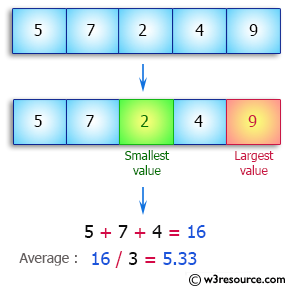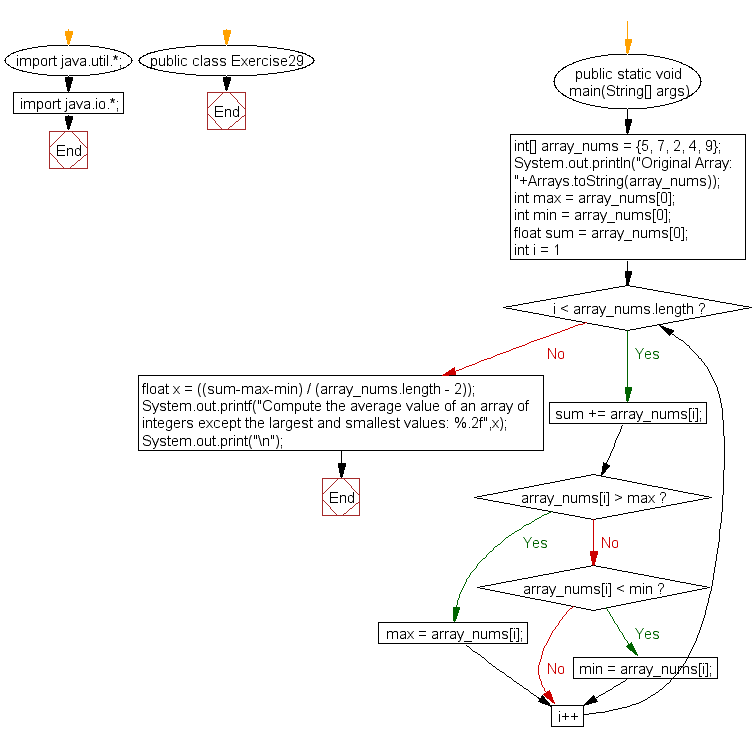﻿ Java: Average value of an array except the largest, smallest# Java Array Exercises: Compute the average value of an array of integers except the largest and smallest values

## Java Array: Exercise-29 with Solution

Write a Java program to compute the average value of an array of integers except the largest and smallest values.

Pictorial Presentation:Sample Solution:

Java Code:

``````import java.util.*;
import java.io.*;
public class Exercise29 {
public static void main(String[] args)
{
int[] array_nums = {5, 7, 2, 4, 9};
System.out.println("Original Array: "+Arrays.toString(array_nums));
int max = array_nums;
int min = array_nums;
float sum = array_nums;
for(int i = 1; i < array_nums.length; i++)
{
sum  += array_nums[i];
if(array_nums[i] > max)
max = array_nums[i];
else if(array_nums[i] < min)
min = array_nums[i];
}
float x = ((sum-max-min) / (array_nums.length - 2));
System.out.printf("Compute the average value of an array of integers except the largest and smallest values: %.2f",x);
System.out.print("\n");
}
}
```
```

Sample Output:

```
Original Array: [5, 7, 2, 4, 9]
Compute the average value of an array of integers except the largest an
d smallest values: 5.33
```

Flowchart:Visualize Java code execution (Python Tutor):

Java Code Editor:

Improve this sample solution and post your code through Disqus

What is the difficulty level of this exercise?

Test your Programming skills with w3resource's quiz.

﻿

## Java: Tips of the Day

getEnumMap

Converts to enum to Map where key is the name and value is Enum itself.

```public static <E extends Enum<E>> Map<String, E> getEnumMap(final Class<E> enumClass) {
return Arrays.stream(enumClass.getEnumConstants())
.collect(Collectors.toMap(Enum::name, Function.identity()));
}
```

Ref: https://bit.ly/3xXcFZt